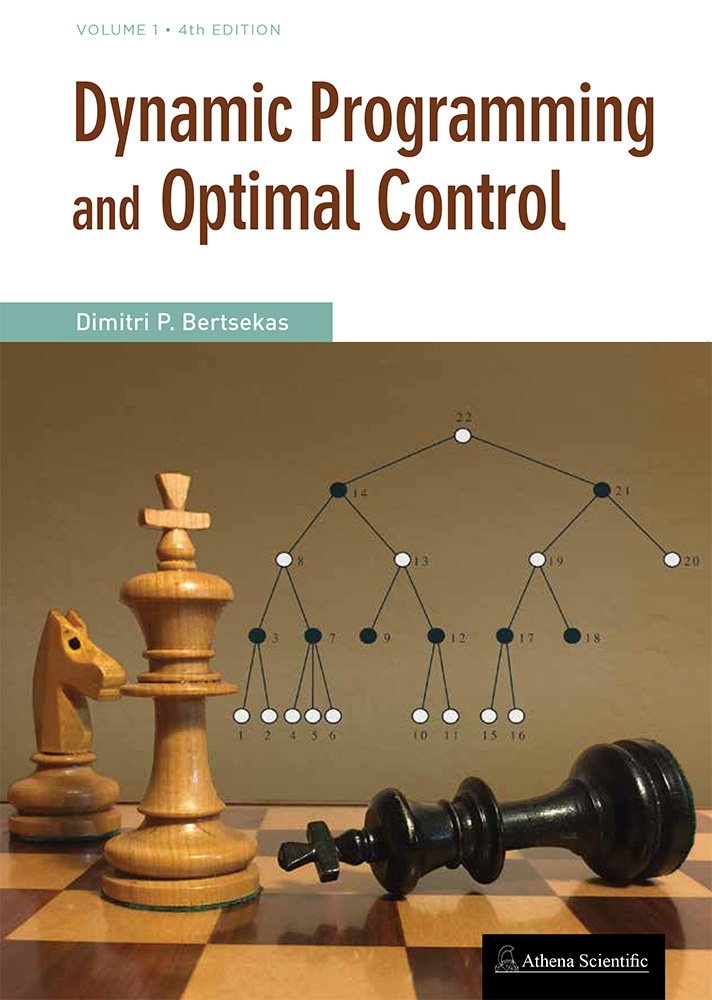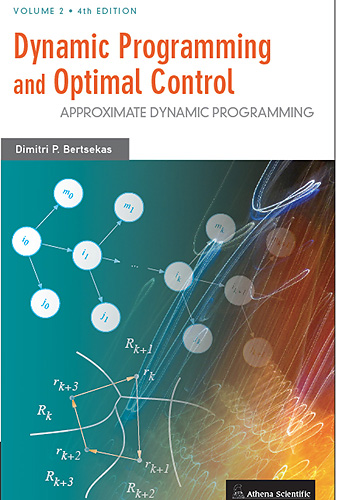# Published February 2017The fourth edition (February 2017) contains a substantial amount of new material, particularly on approximate DP in Chapter 6. This chapter was thoroughly reorganized and rewritten, to bring it in line, both with the contents of Vol. II, whose latest edition appeared in 2012, and with recent developments, which have propelled approximate DP to the forefront of attention.

Some of the highlights of the revision of Chapter 6 are an increased emphasis on one-step and multistep lookahead methods, parametric approximation architectures, neural networks, rollout, and Monte Carlo tree search. Among other applications, these methods have been instrumental in the recent spectacular success of computer Go programs. The material on approximate DP also provides an introduction and some perspective for the more analytically oriented treatment of Vol. II.

Click here for direct ordering from the publisher and preface, table of contents, supplementary educational material, lecture slides, videos, etc

Dynamic Programming and Optimal Control, Vol. I, ISBN-13: 978-1-886529-43-4, 576 pp., hardcover, 2017

# Published June 2012The fourth edition of Vol. II of the two-volume DP textbook was published in June 2012. This is a major revision of Vol. II and contains a substantial amount of new material, as well as a reorganization of old material. The length has increased by more than 60% from the third edition, and most of the old material has been restructured and/or revised. Volume II now numbers more than 700 pages and is larger in size than Vol. I. It can arguably be viewed as a new book!

Approximate DP has become the central focal point of this volume, and occupies more than half of the book (the last two chapters, and large parts of Chapters 1-3). Thus one may also view this new edition as a followup of the author's 1996 book "Neuro-Dynamic Programming" (coauthored with John Tsitsiklis). A lot of new material, the outgrowth of research conducted in the six years since the previous edition, has been included.

A new printing of the fourth edition (January 2018) contains some updated material, particularly on undiscounted problems in Chapter 4, and approximate DP in Chapter 6. References were also made to the contents of the 2017 edition of Vol. I, and to high profile developments in deep reinforcement learning, which have brought approximate DP to the forefront of attention.

Dynamic Programming and Optimal Control, Vol. II: Approximate Dynamic Programming, ISBN-13: 978-1-886529-44-1, 712 pp., hardcover, 2012

## CHAPTER UPDATE - NEW MATERIAL

Click here for an updated version of Chapter 4, which incorporates recent research on a variety of undiscounted problem topics, including

• Deterministic optimal control and adaptive DP (Sections 4.2 and 4.3).

• Stochastic shortest path problems under weak conditions and their relation to positive cost problems (Sections 4.1.4 and 4.4).

• Affine monotonic and multiplicative cost models (Section 4.5).

## PREFACE, SLIDES, AND OTHER INFORMATION

Click here for preface and detailed information.

Lectures on Exact and Approximate Finite Horizon DP: Videos from a 4-lecture, 4-hour short course at the University of Cyprus on finite horizon DP, Nicosia, 2017. Videos from Youtube. (Lecture Slides: Lecture 1, Lecture 2, Lecture 3, Lecture 4.)

Videos from a 6-lecture, 12-hour short course at Tsinghua Univ., Beijing, China, 2014. From the Tsinghua course site, and from Youtube. Click here to download Approximate Dynamic Programming Lecture slides, for this 12-hour video course.

Click here to download lecture slides for a 7-lecture short course on Approximate Dynamic Programming, Caradache, France, 2012.

Click here to download lecture slides for the MIT course "Dynamic Programming and Stochastic Control (6.231), Dec. 2015. The last six lectures cover a lot of the approximate dynamic programming material.

Click here to download research papers and other material on Dynamic Programming and Approximate Dynamic Programming.

Contents:

1. Discounted Problems - Theory

1. Minimization of Total Cost - Introduction
1. The Finite-Horizon DP Algorithm
2. Shorthand Notation and Monotonicity
3. A Preview of Infinite Horizon Results
4. The Finite-Horizon DP Algorithm
5. Randomized and History-Dependent Policies
2. Discounted Problems - Bounded Cost per Stage
3. Scheduling and Multiarmed Bandit Problems
4. Discounted Continuous-Time Problems
5. The Role of Contraction Mappings
1. Sup-Norm Contractions
2. Discounted Problems - Unbounded Cost per Stage
6. General Forms of Discounted Dynamic Programming
1. Basic Results Under Contraction and Monotonicity
2. Discounted Dynamic Games
7. Notes, Sources, and Exercises

2. Discounted Problems - Computational Methods

1. Markovian Decision Problems
2. Value Iteration
1. Monotonic Error Bounds for Value Iteration
2. Variants of Value Iteration
3. Q-Learning
3. Policy Iteration
1. Policy Iteration for Costs
2. Policy Iteration for Q-Factors
3. Optimistic Policy Iteration
4. Limited Lookahead Policies and Rollout
4. Linear Programming Methods
5. Methods for General Discounted Problems
1. Limited Lookahead Policies and Approximations
2. Generalized Value Iteration
3. Approximate Value Iteration
4. Generalized Policy Iteration
5. Generalized Optimistic Policy Iteration
6. Approximate Policy Iteration
7. Mathematical Programming
6. Asynchronous Algorithms
1. Asynchronous Value Iteration
2. Asynchronous Policy Iteration
3. Policy Iteration with a Uniform Fixed Point
7. Notes, Sources, and Exercises

3. Stochastic Shortest Path Problems

1. Problem Formulation
2. Main Results
3. Underlying Contraction Properties
4. Value Iteration
1. Conditions for Finite Termination
2. Asynchronous Value Iteration
5. Policy Iteration
1. Optimistic Policy Iteration
2. Approximate Policy Iteration
3. Policy Iteration with Improper Policies
4. Policy Iteration with a Uniform Fixed Point
6. Countable State Spaces
7. Notes, Sources, and Exercises

4. Undiscounted Problems

1. Unbounded Costs per Stage
1. Main Results
2. Value Iteration
3. Other Computational Methods
2. Linear Systems and Quadratic Cost
3. Inventory Control
4. Optimal Stopping
5. Optimal Gambling Strategies
6. Nonstationary and Periodic Problems
7. Notes, Sources, and Exercises

5. Average Cost per Stage Problems

1. Finite-Spaces Average Cost Models
1. Relation with the Discounted Cost Problem
2. Blackwell Optimal Policies
3. Optimality Equations
2. Conditions for Equal Average Cost for all Initial States
3. Value Iteration
1. Single-Chain Value Iteration
2. Multi-Chain Value Iteration
4. Policy Iteration
1. Single-Chain Policy Iteration
2. Multi-Chain Policy Iteration
5. Linear Programming
6. Infinite-Spaces Problems
1. A Sufficient Condition for Optimality
2. Finite State Space and Infinite Control Space
3. Countable States -- Vanishing Discount Approach
4. Countable States -- Contraction Approach
5. Linear Systems with Quadratic Cost
7. Notes, Sources, and Exercises

6. Approximate Dynamic Programming - Discounted Models

1. General Issues of Simulation-Based Cost Approximation
1. Approximation Architectures
2. Simulation-Based Approximate Policy Iteration
3. Direct and Indirect Approximation
4. Monte Carlo Simulation
5. Simplifications
2. Direct Policy Evaluation - Gradient Methods
3. Projected Equation Methods for Policy Evaluation
1. The Projected Bellman Equation
2. The Matrix Form of the Projected Equation
3. Simulation-Based Methods
4. LSTD, LSPE, and TD(0) Methods
5. Optimistic Versions
6. Multistep Simulation-Based Methods
7. A Synopsis
4. Policy Iteration Issues
1. Exploration Enhancement by Geometric Sampling
2. Exploration Enhancement by Off-Policy Methods
3. Policy Oscillations - Chattering
5. Aggregation Methods
1. Cost Approximation via the Aggregate Problem
2. Cost Approximation via the Enlarged Problem
3. Multistep Aggregation
4. Asynchronous Distributed Aggregation
6. Q-Learning
1. Q-Learning: A Stochastic VI Algorithm
2. Q-Learning and Policy Iteration
3. Q-Factor Approximation and Projected Equations
4. Q-Learning for Optimal Stopping Problems
5. Q-Learning and Aggregation
6. Finite Horizon Q-Learning
7. Notes, Sources, and Exercises

7. Approximate Dynamic Programming - Nondiscounted Models and Generalizations

1. Stochastic Shortest Path Problems
2. Average Cost Problems
1. Approximate Policy Evaluation
2. Approximate Policy Iteration
3. Q-Learning for Average Cost Problems
3. General Problems and Monte Carlo Linear Algebra
1. Projected Equations
2. Matrix Inversion and Iterative Methods
3. Multistep Methods
4. Extension of Q-Learning for Optimal Stopping
5. Equation Error Methods
6. Oblique Projections
7. Generalized Aggregation
8. Deterministic Methods for Singular Linear Systems
9. Stochastic Methods for Singular Linear Systems
4. Approximation in Policy Space
1. The Gradient Formula
2. Computing the Gradient by Simulation
3. Essential Features for Gradient Evaluation
4. Approximations in Policy and Value Space
5. Notes, Sources, and Exercises

8. Appendix A: Measure-Theoretic Issues in Dynamic Programming

1. A Two-Stage Example
2. Resolution of the Measurability Issues